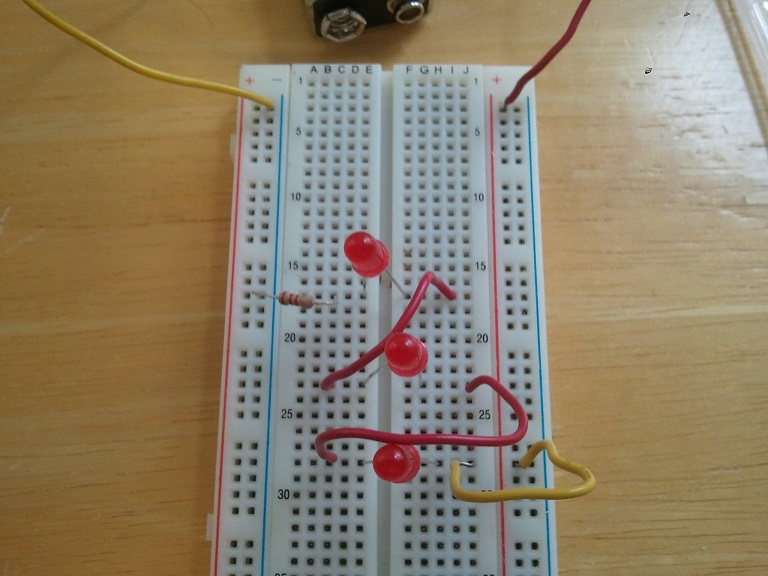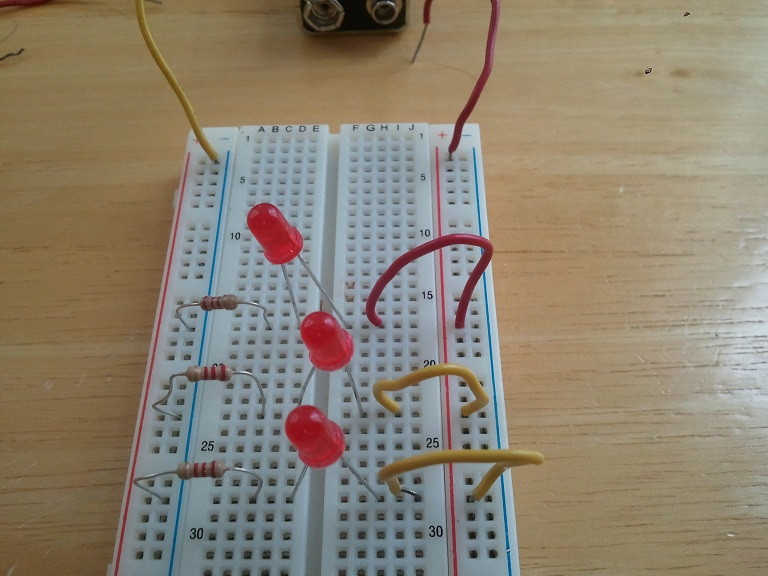# Building a Series and Parallel Circuit

This video will explain the basics of series and prrallel circuits.

Now it's time to build a series circuit but remember...
• the resistor needs to be in the right spot
• the pos (+) and neg (-) of the battery have to be properly matched with the pos (+) and neg (-) of the breadboard
• LEDs have a pos (+) and a neg (-) side, pay close attention to this. The short leg and flat edge is neg(-) while the long leg and rounded side is pos (+).
Build the following SERIES CIRCUIT:Once you have it working, consider the following questions:
1. Why do we only need one resistor, don't we need one for each LED?
2. What happens if we unplug one LED? Why does this happen?
Now it's time to build a parallel circuit but remember...
• the resistor needs to be in the right spot
• the pos (+) and neg (-) of the battery have to be properly matched with the pos (+) and neg (-) of the breadboard
• LEDs have a pos (+) and a neg (-) side, pay close attention to this. The short leg and flat edge is neg(-) while the long leg and rounded side is pos (+).
Build the following PARALLEL CIRCUIT:Once you have it working, consider the following questions:
1. Why do we need one resistor for each LED?
2. What happens if we unplug one LED? Why does this happen?